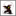Search:
82728 packages online• About • Recent • Browse • Search • Upload • Setup • Services

## misc/math/ci.lha

 Mirror: Random Showing:No screenshot available
 Short: Desktop calculator for complex numbers Author: thomas.radtkeuni-essen.de Uploader: thomas radtke uni-essen de Type: misc/math or misc/edu Replaces: ci.lha Architecture: m68k-amigaos Date: 1997-06-27 Download: http://aminet.net/misc/math/ci.lha - View contents Readme: http://aminet.net/misc/math/ci.readme Downloads: 2112
```new in this release (97/06/26):
some functions, variables, new constants pi and last

Hi #?,

This is ci, the desktop calculator for operations
on complex numbers. To install, just copy it to c: or
anywhere else in your command path. Call it with 'ci'
and leave it by pressing 'Ctrl-\' (control backslash, EOF).

ci accepts inputs in the conventional manner, that
is, real and imaginary part are seperated by addition or
subtraction. The imaginary part is multiplied or divided
by i (square root of -1).

ci knows the following operators:

a+b, a-b, a*b, a/b	-	the usual
a^b			-	exponentiationa			-	mean argument (angle)
|a|			-	modulus
~a			-	complex conjugation

ci knows the following constants:

i			-	square root of -1
e			-	Euler number
pi			-	pi
last			-	value of last expression
(this is a const for technical
reasons only, i.e. the user may
not change it)

ci knows the following functions:

re(z)			-	returns the real part of z
im(z)			-	returns the imaginary part of z
exp(z)
sqrt(z)
sin(z)
cos(z)
sinh(z)
cosh(z)

ci understands assignments to variables (e.g. a1_2=1)

Every trigonometric operation can be acomplished with the
exponentiation operator, e.g.:

sin(x) == (e^(z*i)-e^(-z*i))/(2*i)

Of course, any root can be computed too, e.g.:

n-th root of a == a^(1/n)

Examples:

\$ ci
>-1^(1/2)
6.123032e-17+i*1.000000e+00	<- real part should be 0 !
>2^2
4.000000e+00+i*0.000000e+00
>e^(4*i+2)
-4.829809e+00+i*-5.592056e+00
>|1+i|
1.414214e+00+i*0.000000e+00
>(1+i)
7.853982e-01+i*0.000000e+00
>(2+3*i)*(i^2)
-2.000000e+00+i*-3.000000e+00

The parser for ci was generated by 'bison'.

You may ask for improvements, but please understand that
I didn't have the time to answer all incoming mails immediatley.
Let me know if you are using ci for educational or other purposes
on a regulary (once a week or so) basis.

bye,

Thomas
```

Contents of misc/math/ci.lha
``` PERMSSN    UID  GID    PACKED    SIZE  RATIO     CRC       STAMP          NAME
---------- ----------- ------- ------- ------ ---------- ------------ -------------
[generic]                11945   23616  50.6% -lh5- e760 Jun 26  1997 ci
[generic]                 1106    2069  53.5% -lh5- 5613 Jun 26  1997 ci.readme
---------- ----------- ------- ------- ------ ---------- ------------ -------------
Total         2 files   13051   25685  50.8%            Jun 27  1997
```Aminet © 1992-2019 Urban Müller and the Aminet team. Aminet contact address: <aminetaminet net>